## Introduction

This practical demonstrates use of the samplers argument in lgcp, which you need to use when you have observed points from only a sample of plots in the survey region.

## Setting things up

library(inlabru)
library(INLA)
library(mgcv)
library(ggplot2)
bru_safe_sp(force = TRUE)

## Get the data

data(gorillas, package = "inlabru")

This dataset is a list (see help(gorillas) for details. Extract the the objects you need from the list, for convenience:

nests <- gorillas$nests mesh <- gorillas$mesh
boundary <- gorillas$boundary gcov <- gorillas$gcov

The gorillas data also contains a plot sample subset which covers 60% of the survey region.

sample <- gorillas$plotsample plotdets <- ggplot() + gg(boundary) + gg(sample$plots) +
gg(sample$nests, pch = "+", cex = 4, color = "red") + geom_text(aes(label = sample$counts$count, x = sample$counts$x, y = sample$counts$y)) + labs(x = "Easting", y = "Northing") plot(plotdets)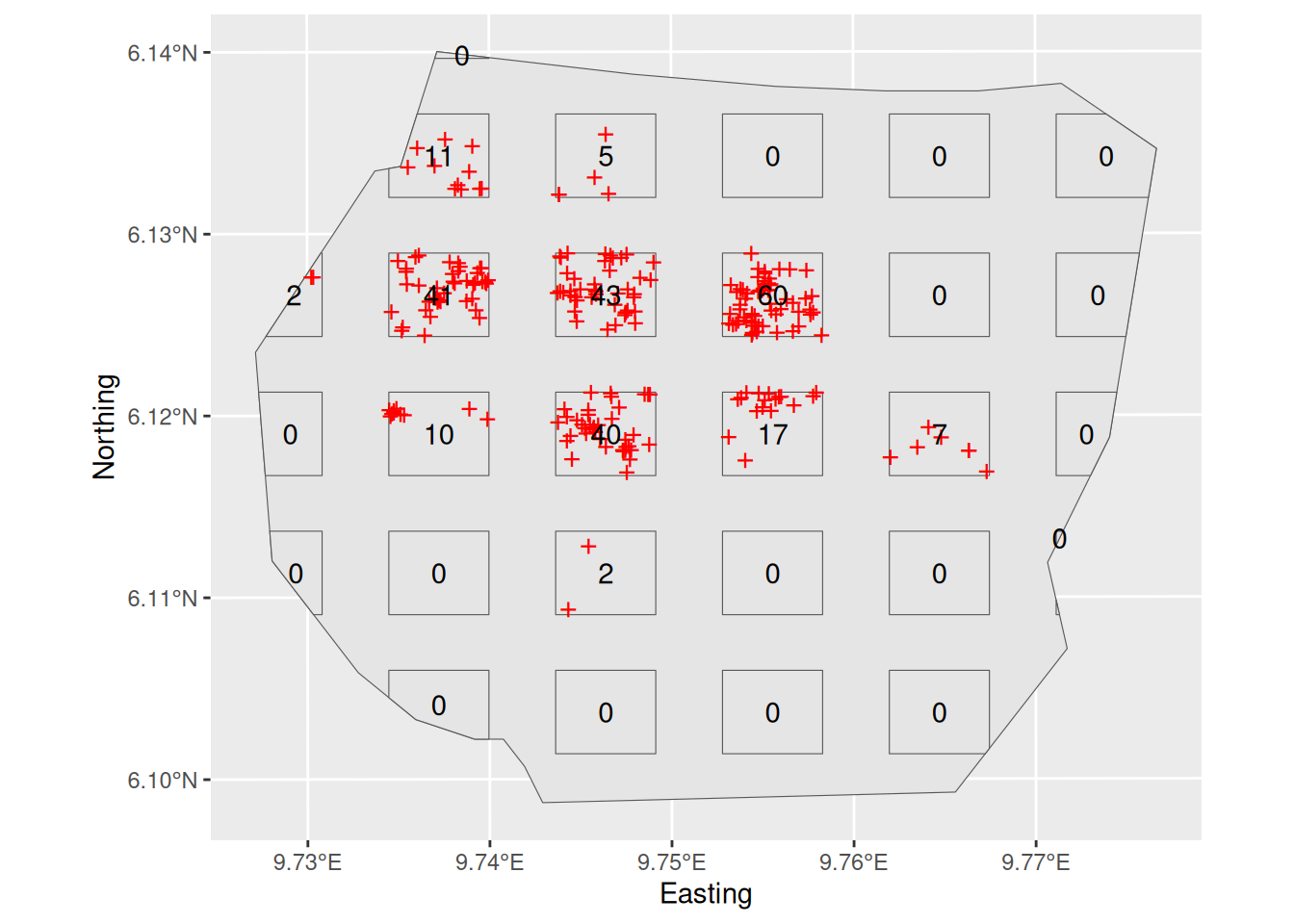On this plot survey, only points within the rectangles are detected, but it is also informative to plot all the points here (which if it was a real plot survey you could not do, because you would not have seen them all). plotwithall <- ggplot() + gg(boundary) + gg(sample$plots) +
gg(nests, pch = "+", cex = 4, color = "blue") +
geom_text(aes(label = sample$counts$count, x = sample$counts$x, y = sample$counts$y)) +
gg(sample$nests, pch = "+", cex = 4, color = "red") + labs(x = "Easting", y = "Northing") plot(plotwithall)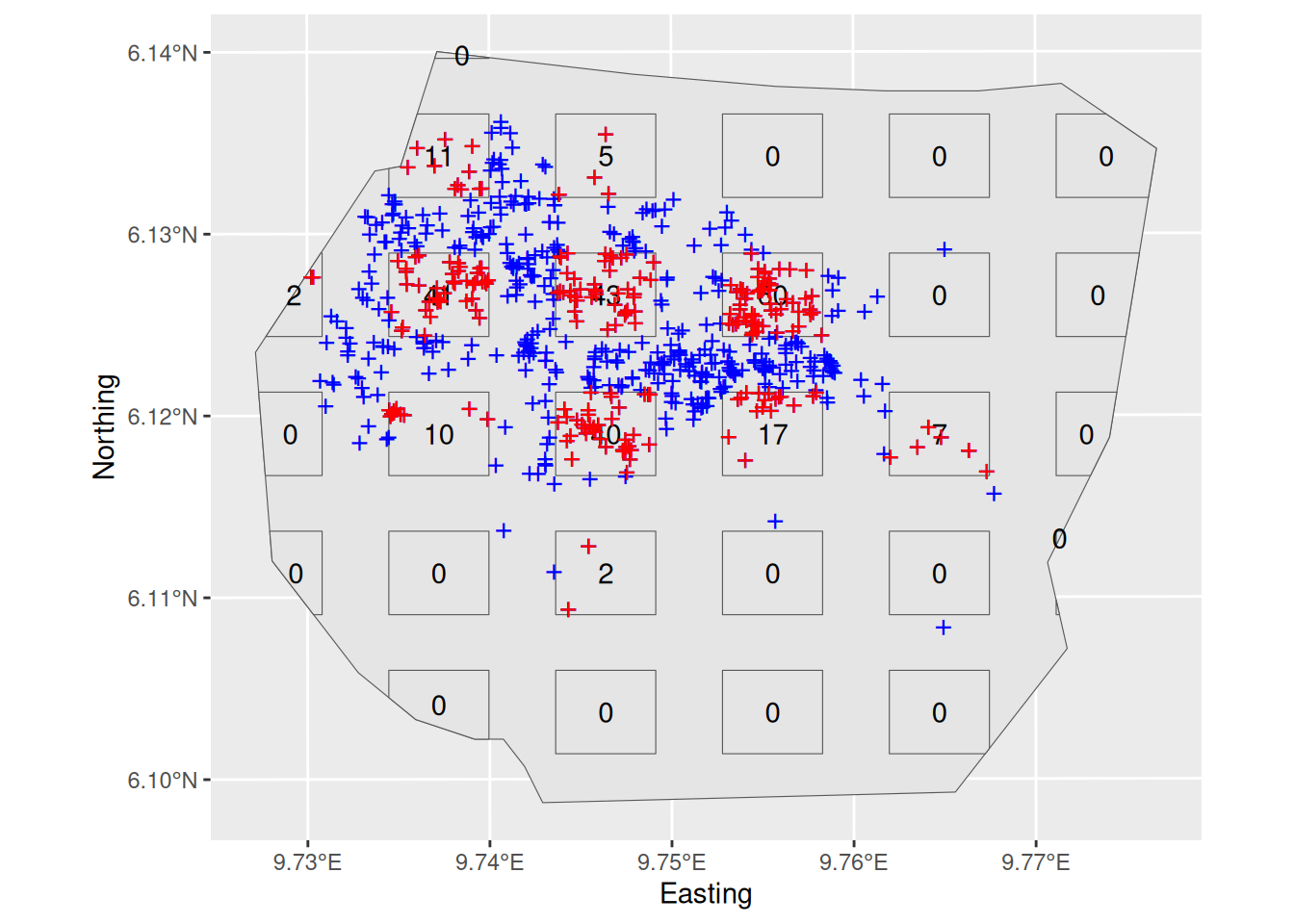## Inference The observed nest locations are in the SpatialPointsDataFrame sample$nests, and the plots are in the SpatialPolygonsDataFrame sample$plots. Again, we are using the following SPDE setup: matern <- inla.spde2.pcmatern(mesh, prior.sigma = c(0.1, 0.01), prior.range = c(0.05, 0.01) ) Fit an LGCP model with SPDE only to these data by using the samplers= argument of the function lgcp( ): cmp <- coordinates ~ my.spde(coordinates, model = matern) fit <- lgcp(cmp, sample$nests, samplers = sample$plots, domain = list(coordinates = mesh)) Plot the density surface from your fitted model pxl <- fm_pixels(mesh, mask = boundary, format = "sp") lambda.sample <- predict(fit, pxl, ~ exp(my.spde + Intercept)) lambda.sample.plot <- ggplot() + gg(lambda.sample) + gg(sample$plots) +
gg(boundary, col = "yellow")

lambda.sample.plot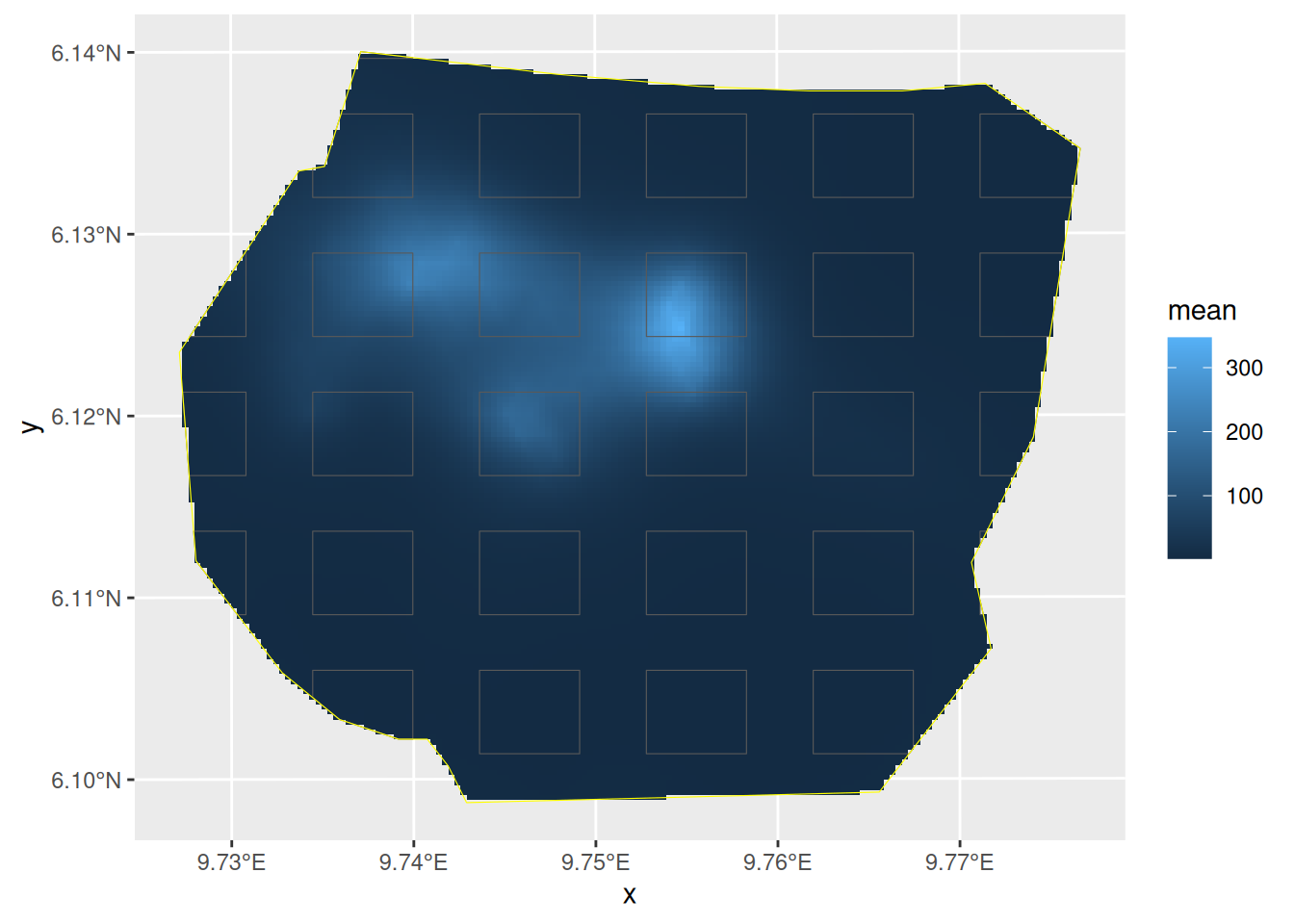Estimate the integrated intensity lambda. We compute both the overall integrated intensity, representative of an imagined new realisation of the point process, and the conditional expectation that takes the actually observed nests into account, by recognising that we have complete information in the surveyed plots.

Lambda <- predict(fit, fm_int(mesh, boundary), ~ sum(weight * exp(my.spde + Intercept)))
Lambda.empirical <- predict(
fit,
rbind(
cbind(fm_int(mesh, boundary), data.frame(all = TRUE)),
cbind(fm_int(mesh, sample$plots), data.frame(all = FALSE)) ), ~ (sum(weight * exp(my.spde + Intercept) * all) - sum(weight * exp(my.spde + Intercept) * !all) + nrow(sample$nests))
)
rbind(
Lambda,
Lambda.empirical
)

Fit the same model to the full dataset (the points in gorillas$nests), or get your previous fit, if you kept it. Plot the intensity surface and estimate the integrated intensity fit.all <- lgcp(cmp, gorillas$nests,
samplers = gorillas$boundary, domain = list(coordinates = mesh) ) lambda.all <- predict(fit.all, pxl, ~ exp(my.spde + Intercept)) Lambda.all <- predict(fit.all, fm_int(mesh, boundary), ~ sum(weight * exp(my.spde + Intercept))) Your plot should look like this: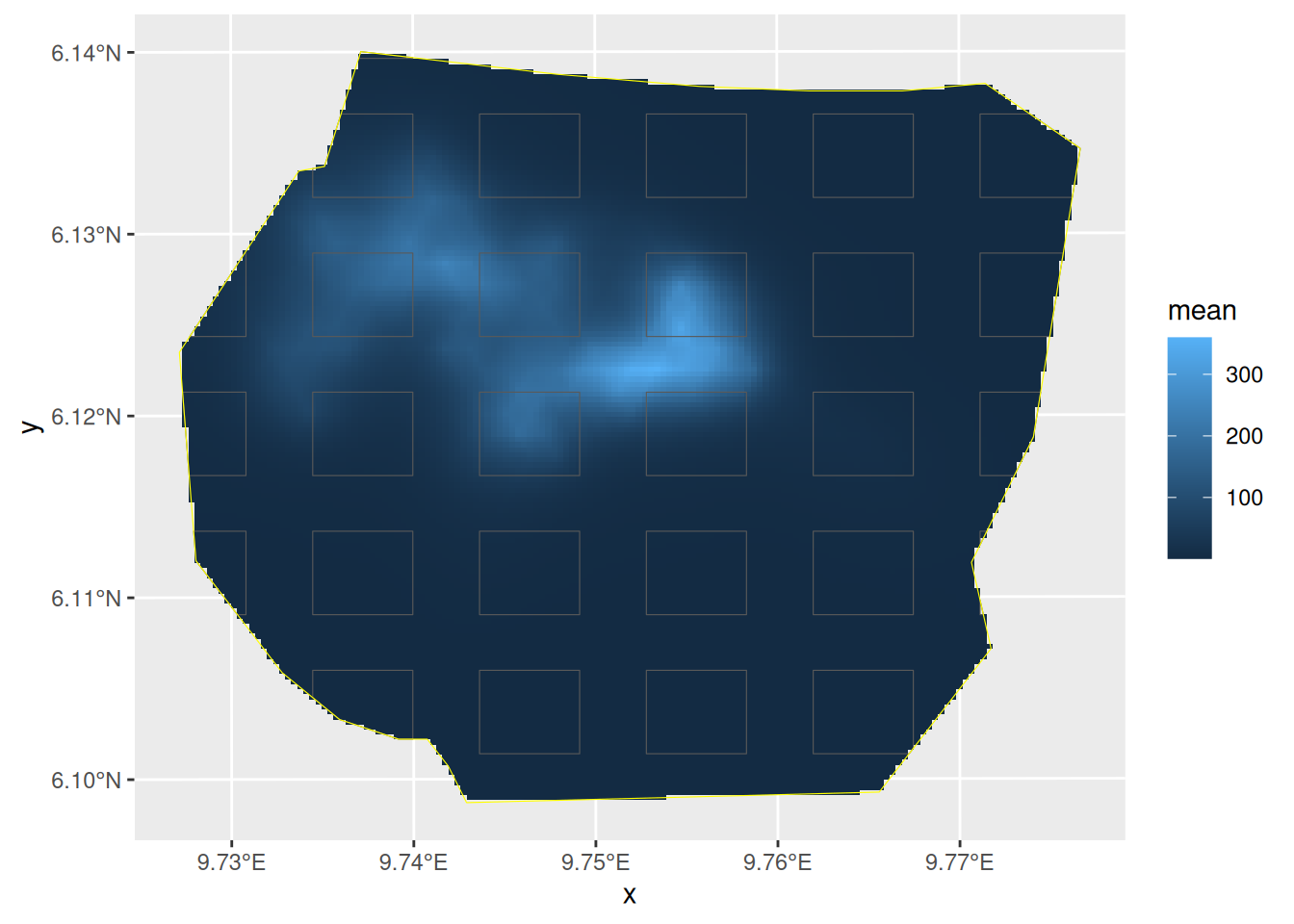The values Lambda.empirical, Lambda, and Lambda.all should be close to each other if the plot samples gave sufficient information for the overall prediction: rbind( Lambda, Lambda.empirical, Lambda.all, Lambda.all.empirical = c(nrow(gorillas$nests), 0, rep(nrow(gorillas\$nests), 3), rep(NA, 4))
)
#> Warning in rbind(deparse.level, ...): number of columns of result, 8, is not a
#> multiple of vector length 9 of arg 4
#>       mean       sd   q0.025     q0.5   q0.975   median mean.mc_std_err
#> 1 660.4464 52.45317 584.6337 650.8880 768.6401 650.8880        5.245317
#> 2 651.5367 39.14195 589.9818 648.1884 732.9213 648.1884        3.914195
#> 3 673.7534 25.97553 625.3560 673.1005 724.5027 673.1005        2.597553
#> 4 647.0000  0.00000 647.0000 647.0000 647.0000       NA              NA
#>   sd.mc_std_err
#> 1      3.948744
#> 2      2.368703
#> 3      1.666048
#> 4            NA

Now, let’s compare the results

library(patchwork)
lambda.sample.plot + lambda.all.plot +
plot_layout(guides = "collect") &
theme(legend.position = "left") &
scale_fill_continuous(limits = range(c(0, 340)))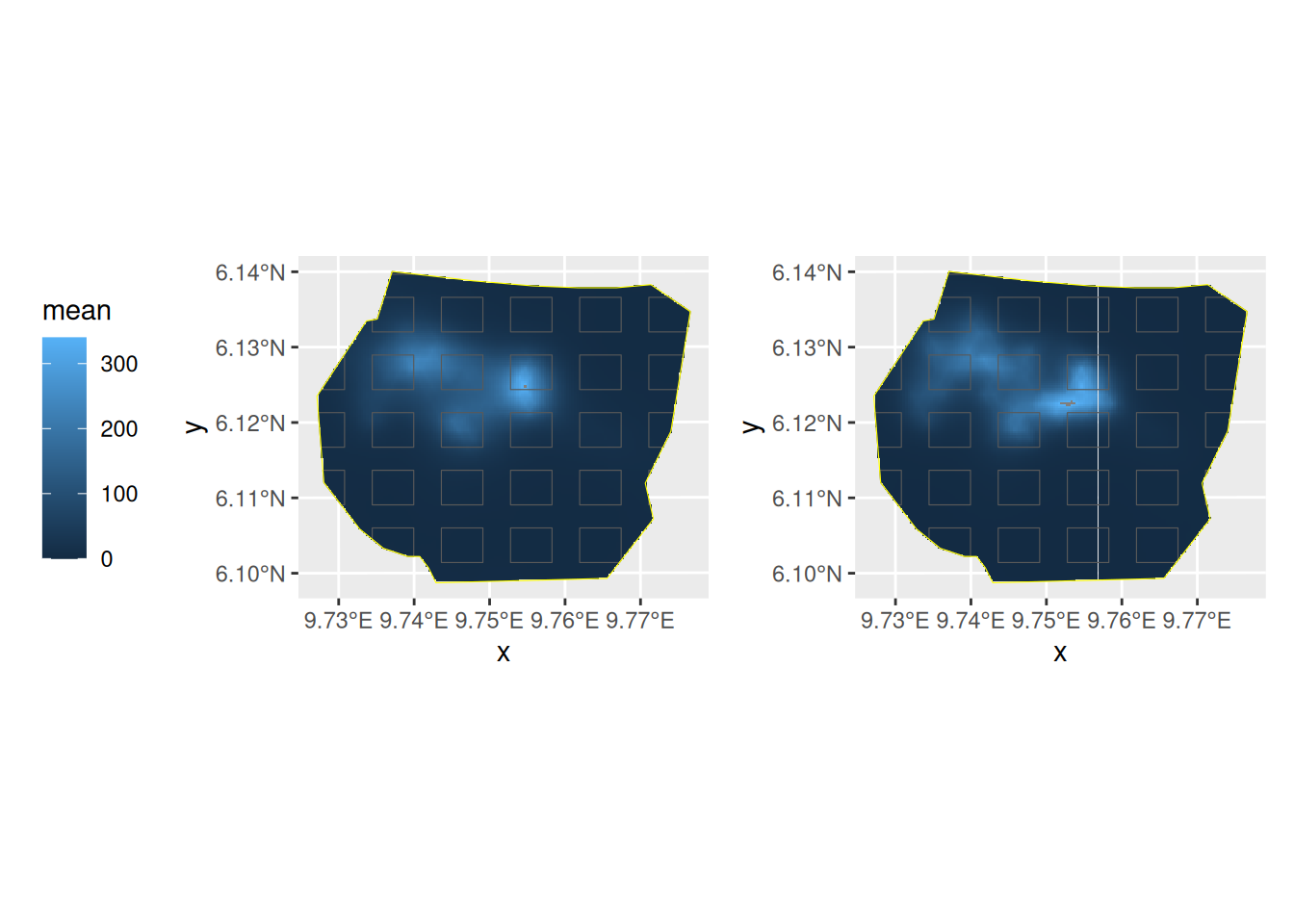Do you understand the reason for the differences in the posteriors of the abundance estimates?